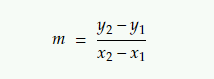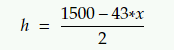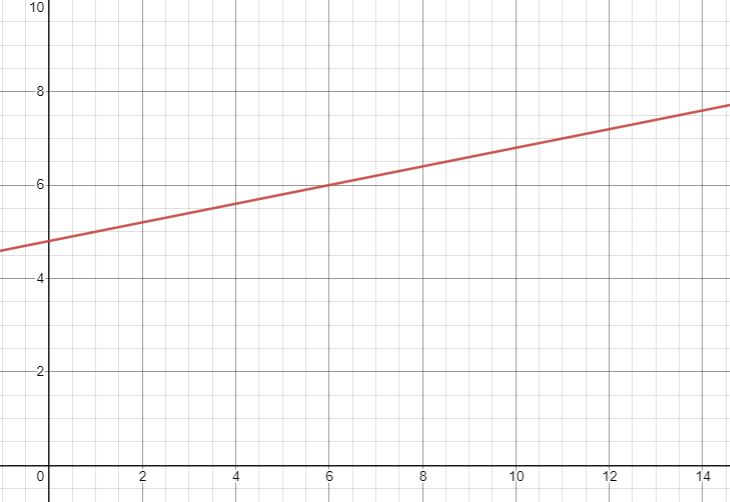# Significance Of Line Parameters On the SAT: Slope Questions

Note: this is part II in a two-part series on the significance of line parameters on the SAT. Part I can be viewed here.

Linear equations are extremely common on the SAT. Among questions on this topic, "line parameter significance" questions are perhaps the most frequent. In these, you're not called upon to calculate anything, but rather to understand the parameters of a line in real-life terms.

By understanding a few simple concepts, you should be able to skip a great deal of text and some confusion if such a question does arise. And you'll be able to answer in a few seconds, a question which would take many other students a great deal of time.

Before discussing this question type, we'll lay out the fundamentals of the line equation, and specifically of slope, which is the topic of our article today. For those who already have a good grasp of the line equation, you can skip to here.Fundamentals of the line equation, and specifically of slope

Let's look at a line:The equation for a line is

y = m*x + b

where m represents the slope of the line, and b represents the y-intercept. When asked to find the equation of a line, you're meant to find these two parameters: 'm' and 'b'. Of course, the parameters can be represented by any random letters. Slope can be represented by 'a' or 's', instead of 'm', for example. It's just a symbol.

As mentioned in previous articles, the purpose of this lesson is not to provide you with a background in line equations. If your knowledge of these is lacking, it might be worth taking some time to sharpen your knowledge in the area. Today's lesson is only about a specific question type likely to appear on the SAT. If you know what to look for, the question can be easily answered without wading through long text, or even knowing much about lines.

We discussed the significance of 'b', the line intercept, in a previous article. Today we'll discuss the significance of the slope, 'm'.

m is the coefficient of x - that is, it's the number being multiplied by x.

m is slope: that is, how quickly the line is increasing. Finding slope:In the above graph, the easiest points to take in this case would be (0, 5) and (5, 15). Plugging these points into the formulas, we'll get:Note that the bigger the absolute value of the slope, the steeper it will be. The lower the absolute value of the slope, the flatter it will be.

In the following graph, the equation for the blue line is: y = 5x + 5

The equation for the red line: y = x + 5For the blue line, the slope is 5. (That's the number being multipled by x.)

For the red line, the slope is 1. (x = 1*x)

The slope of the blue line (5) is numerically greater than that of the red one (1). As you can see on the graph, the blue line is correspondingly steeper. For each unit of x, the blue rises much more than the red line does for the same unit of x.

Look at what happens when x=1. When x=0, the two lines are at the same value - that is, 5. But when x=1, the red line is at 6, and the blue line is already at 10.

When m is negative, the line slopes downwards. In practical terms, this means that the function is decreasing. For every unit we move in the x direction, the less we have of whatever we're measuring.

As an example, let's say that the function y = 10 - 2x represents the amount of pocket money a child has. \$10.00 is the amount the child receives at the beginning of the week, and x represents the number of days which have passed since then. Note that the m here is negative: m = -2. This indicates that the amount of the child's pocket money is decreasing with each passing day. (Since, presumably, she's spending it.)

The following is a graph of the above equation. Note how the slope is decreasing:The physical significance of the slope parameter

Slope signifies how much the function is increasing or decreasing for every unit of your independent variable x. Unless the line is totally flat, it will do one of the two.

Take as an example the equation representing the child's pocket money: y = 10 - 2*x. The slope, as derived from the line equation, is -2, meaning that the child loses \$2 per day.The word 'per' is important here, since it's one of the keywords indicative of slope.

Keywords for slope

Just as with the y-intercept, there are certain words indicative of slope. Below are some examples:

• Increasing, decreasing

• rate, pace

• per, for every, for each

• daily, monthly, yearly, etc.

'Daily', 'monthly', etc are on the list because they're a way of saying 'PER' month, 'PER' day, without using the word 'per.'

Now let's look at some examples.

Examples

Example #1

The following equation models the number of sea turtles in mating season, on a specific beach of the Gulf Islands, between May and September:

y = 110 + 15*d

where d is the number of days from May 1.

Question #1 Based on the model, what’s the estimated increase in the number of sea turtles each day?

A) 110

B) 15

C) 125

D) 30'Increase' and 'each' are both words indicative of slope. The slope in the equation - that is, the coefficient of d - is 15. The answer is B).

'd' here is used as the x parameter. Remember that the specific letters used to represent familiar concepts, is irrelevant.

Example #2

The relationship between the total cost, y, of restaurant purchase in terms of food expenses, upkeep, and electricity, and the number of employees the restaurant utilizes, x, is modeled by the equation y = a*x + b.

Question #2

What is represented by the slope of the graph in this equation?

A) The total daily cost of upkeep

B) The total daily cost of running the restaurant

C) The total cost of maintaining the restaurant

D) The total cost for food expenses.Glancing at the answer options, we can see that A) and B) use a slope keyword: 'daily.' The answer must be from among these two.

Slope indicates how the function y is increasing each day. y here represents total costs, not costs for upkeep alone, food expenses alone, or any other individual expenses. The answer is B).

Example #3

The heart rate h, in beats per minute, of mammals as a function of their average life expectancy, x, in years, can be modeled by the equation:Question #3

For every increase of 1 year in average life expectancy, by how much will the average heart rate decrease in beats per minute?

A) 1500

B) 43

C) 21.5

D) 1457Firstly, this is a linear equation. It may not look like it, but realize that if you divide both elements by 2, that's exactly what you'll get:The question's wording is convoluted, but don't let that intimidate you. The question is a linear question. Look at what they're asking in the question, the keywords they're using: 'every increase of 1 year', 'average heart rate decrease'. They're just asking for the slope.

The heart rate is increasing by -21.5, or decreasing by 21.5. The answer is C).

Example #4

During each month of the year, scientists collected information on the bersha bird in the south Congo. They measured the amount of oki seeds the population of bersha birds ate during each month. The scientists discovered that the amount of oki seeds the birds ate every month could be fitted to a linear graph. The equation for the line graph they found was y = 4.8 + 0.2*x, where y is the amount of oki seeds the bersha birds ate each month, measured in millions of kilograms, and x is the number of months passed since the beginning of the year.

Below is the graph of the representative equation:Question #4

What does 0.2 represent in the equation given above?

A) The total amount of oki seeds, in millions of kilograms, eaten by the bersha birds in the first month

B) The average monthly amount of oki seeds, in millions of kilograms, eaten by the bersha birds.

C) The average monthly increase in oki seeds, in millions of kilograms, eaten by the bersha birds.

D) The average time it takes for the bersha birds to eat 4.8 millions of kilograms of oki seeds.0.2 is the slope in this equation. The only answer with the appropriate keywords is C): 'The average monthly increase in oki seeds, in millions of kilograms, eaten by the bersha birds.'

Note that the above question, like many of the questions before it, has a plethora of extra detail meant to disguise the fact that this is an easy question. In this particular case, the graph is one of those extra details: you don't need it to answer.

Note that the only reason you could need a graph for such questions is if they didn't give you the equation, and you so needed to figure out the slope from the graph. You need one or the other. Here, they gave you the equation and thus the graph. Move on.

Now let's look at an example which welds the lessons we learned in part 1 of this topic, regarding y intercepts, with the lessons we saw in this article.

Example #5

Mindy is scaling a mountain. She starts at an area 55 feet above the ground, and begins climbing at a rate of 7 feet per minute.

Question #5

If y represents Mindy's height, in feet, and x represents the number of minutes that have passed since Mindy starts to climb, which of the following equations would represent Mindy's height?

A) y = 55 +7x

B) y = 7+55x

C) y = x + (55/7)

D) y = 1 + (55/7)*xIf you've read the article on y-intercept, you know that "starts at" is a keyword for y-intercept. As such, 55 is the y-intercept in this equation. Similarly, "rate of" and "per minute" are keywords for slope, meaning that 7 is the slope in this equation. So the correct equation here would be y = 55 + 7*x.

Conclusion

The existence of questions like these doesn't mean that you won't be asked to calculate slope on SAT questions. In all likelihood, you will be. There's a plethora of linear equations on the SAT, and many of them require actual calculations or understanding of the physical situation. But remember: when you see a question where either the linear equation is given explicitly, or the line is given in a way where the y-intercept or slope is easily visible, check if the question is really, in one way or another, asking for one of the parameters. If so, answer the question and move on.

For more tips and methods, or to get help personally tailored to your needs, consider working with me. I've helped people from all over the world get into their dream school. In coaching you, I adopt my methods specifically to your personality, schedule, and learning style. For this reason, studying privately with a skilled coach is the best way to increase your test score.

Happy learning,

Tova

Tags: